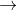In: Chemistry

# If a solution containing 17.91 g17.91 g of mercury(II) perchlorate is allowed to react completely with...

If a solution containing 17.91 g17.91 g of mercury(II) perchlorate is allowed to react completely with a solution containing 5.102 g5.102 g of sodium dichromate, how many grams of solid precipitate will be formed?

mass:

gg

How many grams of the reactant in excess will remain after the reaction?

mass:

gg

Assuming complete precipitation, how many moles of each ion remain in solution? If an ion is no longer in solution, enter a zero (0) for the number of moles.

Hg2+Hg2+:

molmol

ClO–4ClO4–:

molmol

Na+Na+:

molmol

Cr2O2−7Cr2O72−:

mol

## Solutions

##### Expert Solution

mass of solid precipitate formed = 8.113 g

mass of reactant in excess = 10.13 g

Hg2+ = 0.02536 mol

ClO42- = 0.08966 mol

Na+ = 0.03895 mol

Cr2O72- = 0 mol

Explanation

Balanced equation : Hg(ClO4)2 + Na2Cr2O7HgCr2O7 + 2 NaClO4

Case 1 : Hg(ClO4)2 is the limiting reactant

mass Hg(ClO4)2 reacted = 17.91 g

moles Hg(ClO4)2 reacted = (mass Hg(ClO4)2 reacted) / (molar mass Hg(ClO4)2)

moles Hg(ClO4)2 reacted = (17.91 g) / (399.49 g/mol)

moles Hg(ClO4)2 reacted = 0.0448 mol

moles HgCr2O7 formed = (moles Hg(ClO4)2 reacted) * (1 mole HgCr2O7 / 1 mole Hg(ClO4)2)

moles HgCr2O7 formed = (0.0448 mol) * (1 / 1)

moles HgCr2O7 formed = 0.0448 mol

mass HgCr2O7 formed = (moles HgCr2O7 formed) * (molar mass HgCr2O7)

mass HgCr2O7 formed = (0.0448 mol) * (416.58 g/mol)

mass HgCr2O7 formed = 18.68 g

Case 2 :

Na2Cr2O7 is the limiting reactant

mass Na2Cr2O7 reacted = 5.102 g

moles Na2Cr2O7 reacted = (mass Na2Cr2O7 reacted) / (molar mass Na2Cr2O7)

moles Na2Cr2O7 reacted = (5.102 g) / (261.97 g/mol)

moles Na2Cr2O7 reacted = 0.01947 mol

moles HgCr2O7 formed = (moles Na2Cr2O7 reacted) * (1 mole HgCr2O7 / 1 mole Na2Cr2O7)

moles HgCr2O7 formed = (0.01947 mol) * (1 / 1)

moles HgCr2O7 formed = 0.01947 mol

mass HgCr2O7 formed = (moles HgCr2O7 formed) * (molar mass HgCr2O7)

mass HgCr2O7 formed = (0.01947 mol) * (416.58 g/mol)

mass HgCr2O7 formed = 8.113 g

Since less mass of HgCr2O7 is formed in Case 2, therefore, Na2Cr2O7 is the limiting reactant.

mass HgCr2O7 (precipitate) formed = 8.113 g

## Related Solutions

##### If a solution containing 37.525 g of mercury(II) perchlorate is allowed to react completely with a...
If a solution containing 37.525 g of mercury(II) perchlorate is allowed to react completely with a solution containing 12.026 g of sodium dichromate, how many grams of solid precipitate will be formed? How many grams of the reactant in excess will remain after the reaction?
##### If a solution containing 84.096 g of mercury(II) perchlorate is allowed to react completely with a...
If a solution containing 84.096 g of mercury(II) perchlorate is allowed to react completely with a solution containing 13.180 g of sodium sulfide, how many grams of solid precipitate will be formed? How many grams of the reactant in excess will remain after the reaction?
##### If a solution containing 63.411 g of mercury(II) perchlorate is allowed to react completely with a...
If a solution containing 63.411 g of mercury(II) perchlorate is allowed to react completely with a solution containing 16.642 g of sodium dichromate, how many grams of solid precipitate will be formed?
##### If a solution containing 39.544 g of mercury(II) perchlorate is allowed to react completely with a...
If a solution containing 39.544 g of mercury(II) perchlorate is allowed to react completely with a solution containing 13.180 g of sodium dichromate, how many grams of solid precipitate will be formed? How many grams of the reactant in excess will remain after the reaction?
##### If a solution containing 16.38 g of mercury (II) nitrate is allowed to react completely with...
If a solution containing 16.38 g of mercury (II) nitrate is allowed to react completely with a solution containing 51.02 g of sodium sulfate, how many grams of solid precipitate will be formed? How many grams of the reactant in excess will remain after the reaction?
##### If a solution containing 42.33 g of mercury(II) chlorate is allowed to react completely with a...
If a solution containing 42.33 g of mercury(II) chlorate is allowed to react completely with a solution containing 6.256 g of sodium sulfide, how many grams of solid precipitate will form? How many grams of the reactant in excess will remain after the reaction?
##### If a solution containing 51.928 g of mercury(II) nitrate is allowed to react completely with a...
If a solution containing 51.928 g of mercury(II) nitrate is allowed to react completely with a solution containing 16.642 g of sodium dichromate, how many grams of solid precipitate will be formed? How many grams of the reactant in excess will remain after the reaction?
##### If a solution containing 78.296 g of mercury(II) acetate is allowed to react completely with a...
If a solution containing 78.296 g of mercury(II) acetate is allowed to react completely with a solution containing 13.180 g of sodium sulfide, how many grams of solid precipitate will be formed? How many grams of the reactant in excess will remain after the reaction?
##### If a solution containing 22.90 g of mercury(II) nitrate is allowed to react completely with a...
If a solution containing 22.90 g of mercury(II) nitrate is allowed to react completely with a solution containing 7.410 g of sodium sulfate, how many grams of solid precipitate will be formed?   How many grams of the reactant in excess will remain after the reaction?
##### If a solution containing 35.10 g of mercury(II) chlorate is allowed to react completely with a...
If a solution containing 35.10 g of mercury(II) chlorate is allowed to react completely with a solution containing 6.256 g of sodium sulfide, how many grams of solid precipitate will be formed? How many grams of the reactant in excess will remain after the reaction?